Tips & Tricks: Mensuration

# Tips & Tricks: Mensuration - Tips & Tricks for Government Exams - Bank Exams

## Introduction

The Mensuration Methods of measuring of geometric magnitudes, lengths, areas, and volumes using mensuration in its meaning is to measure, its normally used where geometrical figures are thoughtful, and where one has to determine various physical quantities such as area, volume, length measuring these quantities is called mensuration it also used where measure quantities like peed.### Mensuration Methods

Now we will discuss some basic ideas of Mensuration Methods. On the basis of these ideas we will learn trick and tips of shortcut mensuration methods. If you think that how to solve mensuration methods questions using mensuration methods shortcut tricks, then further studies will help you to do so.

• Square
A square which has all sides equal and all the four angles of square has equal and to 90º.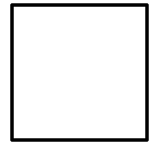• Arc of a Circle
In a circle a curved part or portion is called Arc of a circle.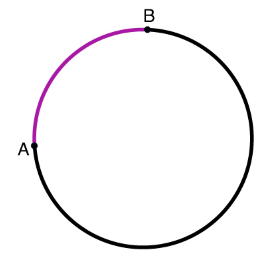• Rectangle
A rectangle with opposite sides are equal and all the four angles are equal to 90º.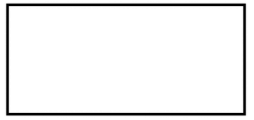• Isosceles Triangle
A triangle which has any two sides equal.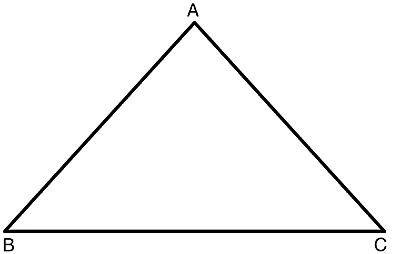Triangle ABC whose AB = AC.
• Equilateral Triangle
A triangle with all sides equal and each angle has 60º which means equal.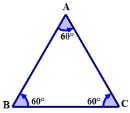### Important Terms

1. Square
In a geometry, Square is a regular quadrilateral and This means that it has four equal sides and four equal angles Each angle is holds 90-degree angles, or right angles of each facing side is equal to the opposite side.
Formula:
(a) Area of Square = 4L2 or (side)2 = 1/2 = (Diagonal)2.
The area can also be calculated using the diagonal d according to
A = D2/2
(b) Perimeter of a Square = 4L or 4 x Side.
The circumference R, the area of a square is
A = 2R2
(c) A room has four wall and its Area of 4 wall is 2 x (Length + Breadth) x Height.

2. Circle
A circle is a simple form and it is a set of series of all points in a plane that is given and its distance make same from the center.
Formula:
(a) Area of a circle
Area of a circle is = πr2
Where π = 22 / 7, or π = 3.14285………
Or Area of Circle is = 22 / 7 x r x r.
(b) Circumference of a Circle
Circumference of a Circle is = 2πr
OR, 2 x 22 / 7 x r (r = radius).
(c) Semi-Circle:
Area of semi-circle = πr2 / 2 = 1 / 2πr2
Circumference = 2πr / 2 + 2r = r(π + 2)
Diameter : = 2 √(area /π)
Area = π (diameter / 2)2
Perimeter = π (diameter).
Diameter = (Perimeter / π).

3. Rectangle
A rectangle is four side of with right angle. Each side of Rectangle is equal and parallel and each angles is a 90° is called Rectangle, and its properties are  given as, Equal angle means that all of its angles are equal ( 360° / 4 = 90° ). It can also be defined as a parallelogram which has containing a right angle.
Formula:
(a) Area of Rectangle
L x B
Here, L = Length and B = Breadth of a Rectangle.
(b) Perimeter of Rectangle
2 x (L + B)

### Solved Examples

Question for Tips & Tricks: Mensuration
Try yourself:

A slice from a circular pizza of diameter 14 inches is cut in a such a way that each slice of pizza has a central angle of 45°. What is the area of each slice of Pizza(in square inches)?

Question for Tips & Tricks: Mensuration
Try yourself:

A rectangular courtyard 3.78 m long and 5.25 m broad is to be paved exactly with square tiles, all of the same size. What will be the minimum number of such tiles is?

Question for Tips & Tricks: Mensuration
Try yourself:

Circumference of a circle A is 22/7 times perimeter of a square. Area of the square is 784 cm². What is the area of another circle B whose diameter is half the radius of the circle A(in cm²)?

The document Tips & Tricks: Mensuration | Tips & Tricks for Government Exams - Bank Exams is a part of the Bank Exams Course Tips & Tricks for Government Exams.
All you need of Bank Exams at this link: Bank Exams

## Tips & Tricks for Government Exams

66 videos|65 docs

## FAQs on Tips & Tricks: Mensuration - Tips & Tricks for Government Exams - Bank Exams

 1. What is mensuration?Ans. Mensuration is a branch of mathematics that deals with the measurement of geometric figures and their parameters such as area, volume, length, and angles.
 2. How is the area of a rectangle calculated?Ans. The area of a rectangle is calculated by multiplying its length by its width. The formula for the area of a rectangle is A = length × width.
 3. What is the formula for calculating the volume of a cylinder?Ans. The formula for calculating the volume of a cylinder is V = πr²h, where V represents the volume, r is the radius of the base, and h is the height of the cylinder.
 4. How do you find the circumference of a circle?Ans. The circumference of a circle can be found using the formula C = 2πr, where C represents the circumference and r is the radius of the circle.
 5. How is the surface area of a sphere calculated?Ans. The surface area of a sphere is calculated using the formula A = 4πr², where A represents the surface area and r is the radius of the sphere.

## Tips & Tricks for Government Exams

66 videos|65 docsExplore Courses for Bank Exams examSignup to see your scores go up within 7 days! Learn & Practice with 1000+ FREE Notes, Videos & Tests.
10M+ students study on EduRev
Track your progress, build streaks, highlight & save important lessons and more!
Related Searches

,

,

,

,

,

,

,

,

,

,

,

,

,

,

,

,

,

,

,

,

,

;